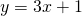# Skill 4 Solve Equations

First, determine whether it is the place value or the term value you need to calculate.

# Skill 5 Two Known Terms

You may have noticed that arithmetic sequences have much in common with straight line graphs.

Compare the sequencewith the straight line.

In a sequence, the valueis usually a natural number– that is, a number from the set. In the linear relation,is a real number – that is, any number at all that you could measure with – a fraction\decimal or whole number or square root and so on.

If you are given two points on a straight line, or if you are given two terms in an arithmetic sequence, you can calculate the equation.

The best algebraic technique for you (the one that makes most sense initially) is often the one that you arrive at yourself. Try the applet, and describe any method you come up with!